# Swift奇趣漫談

## 一、前言

Swift是完全開源的語言，Swift主頁地址，主要採用C++編寫，Swift編譯過程如下：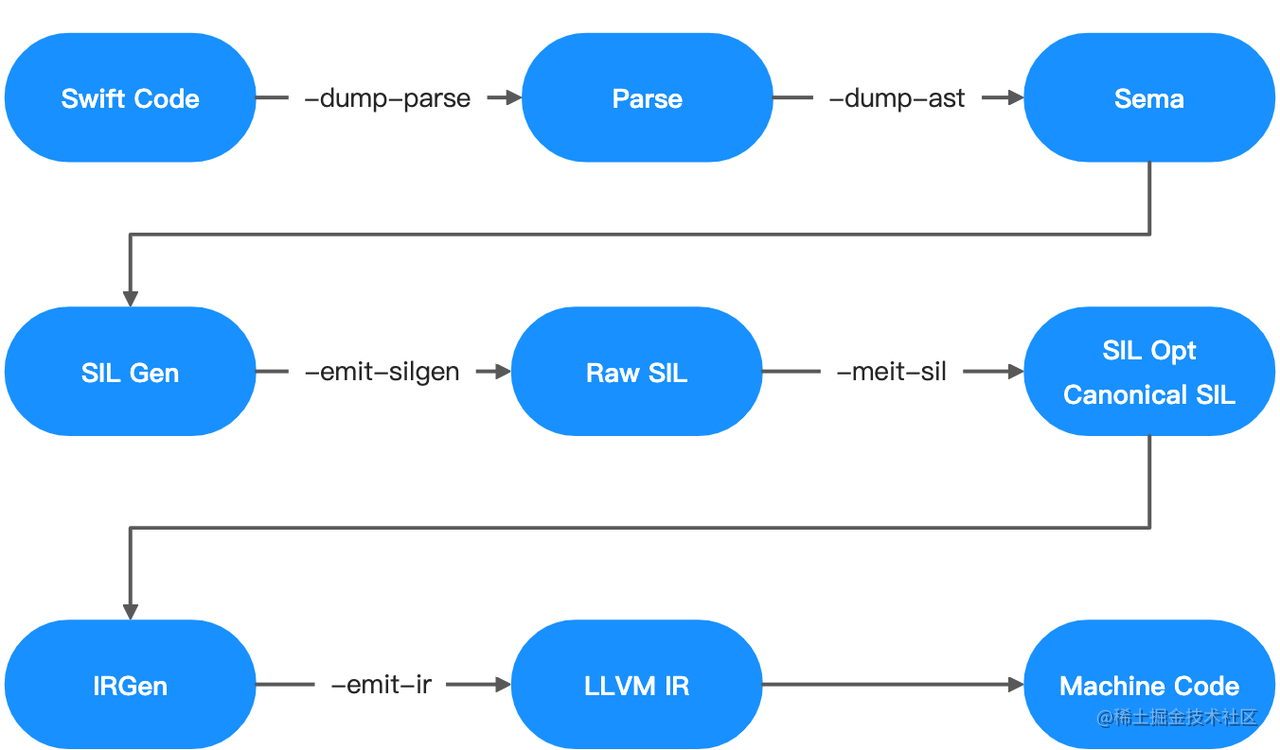# 生成語法樹

swiftc -dump-ast main.swift

# 生成最簡介的SIL程式碼

swiftc -emit-sil main.swift

# 生成LLVM IR程式碼

swiftc -emit-ir main.swift -o main.ll

# 生成彙編程式碼

swiftc -emit-assembly main.swift -o main.s ```

## 二、彙編和除錯

### 2.1 暫存器和記憶體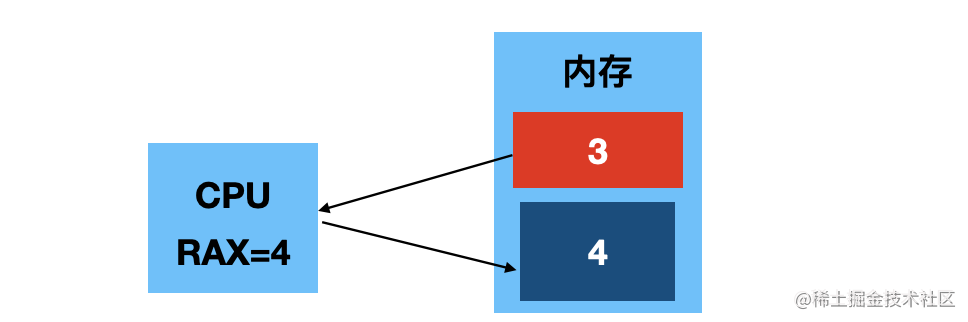### 2.2 彙編基礎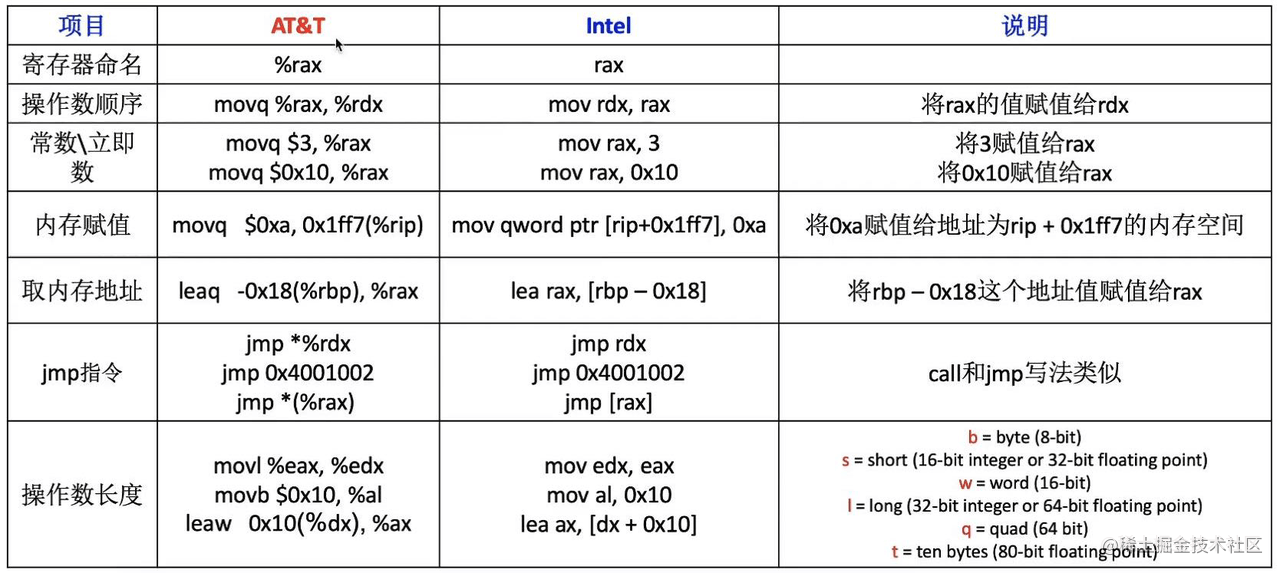# 將 rbp-0x18 指向記憶體地址的值，取出來賦值給 rax

movq -0x18(%rbp), %rax

# 將 rbp-0x18 指向記憶體地址，直接賦值給 rax

leaq -0x18(%rbp), %rax ```

rip作為指令指標： - 儲存著CPU下一條要執行的指令的地址 - 一旦CPU讀取一條指令、rip會自動指向下一條指令（儲存下一條指令的地址）

`Xcode`打斷點，進入彙編顯示頁面的方法： Xcode選單欄-->Debug-->Debug Workflow-->Always Show Disassembly

### 2.3 LLDB常用指令

LLDB是Xcode預設的偵錯程式。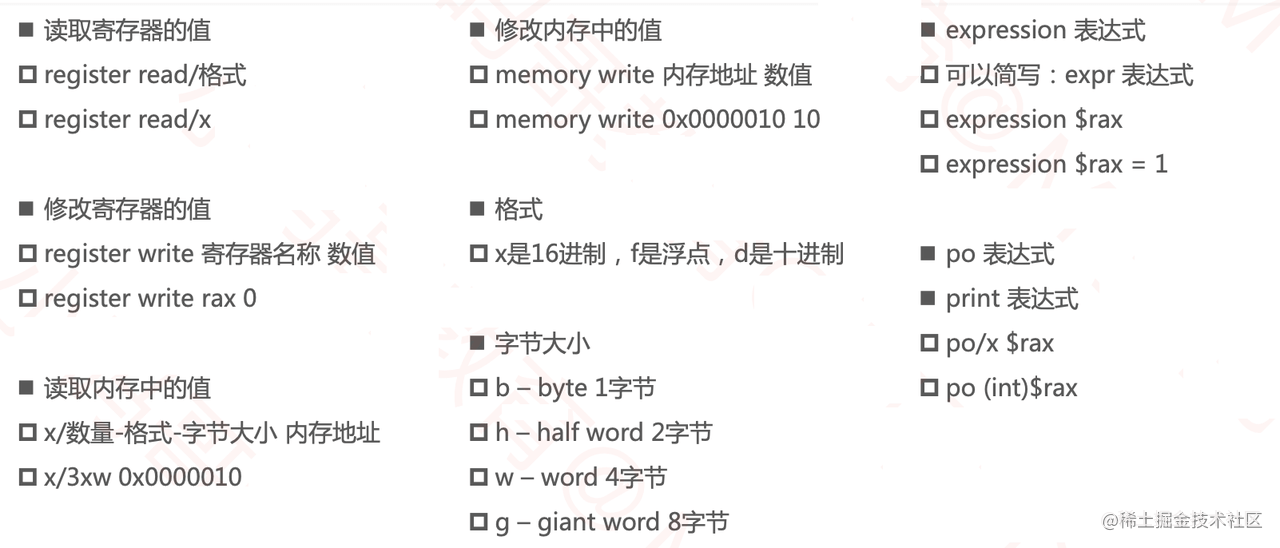### 2.4 LLDB斷點除錯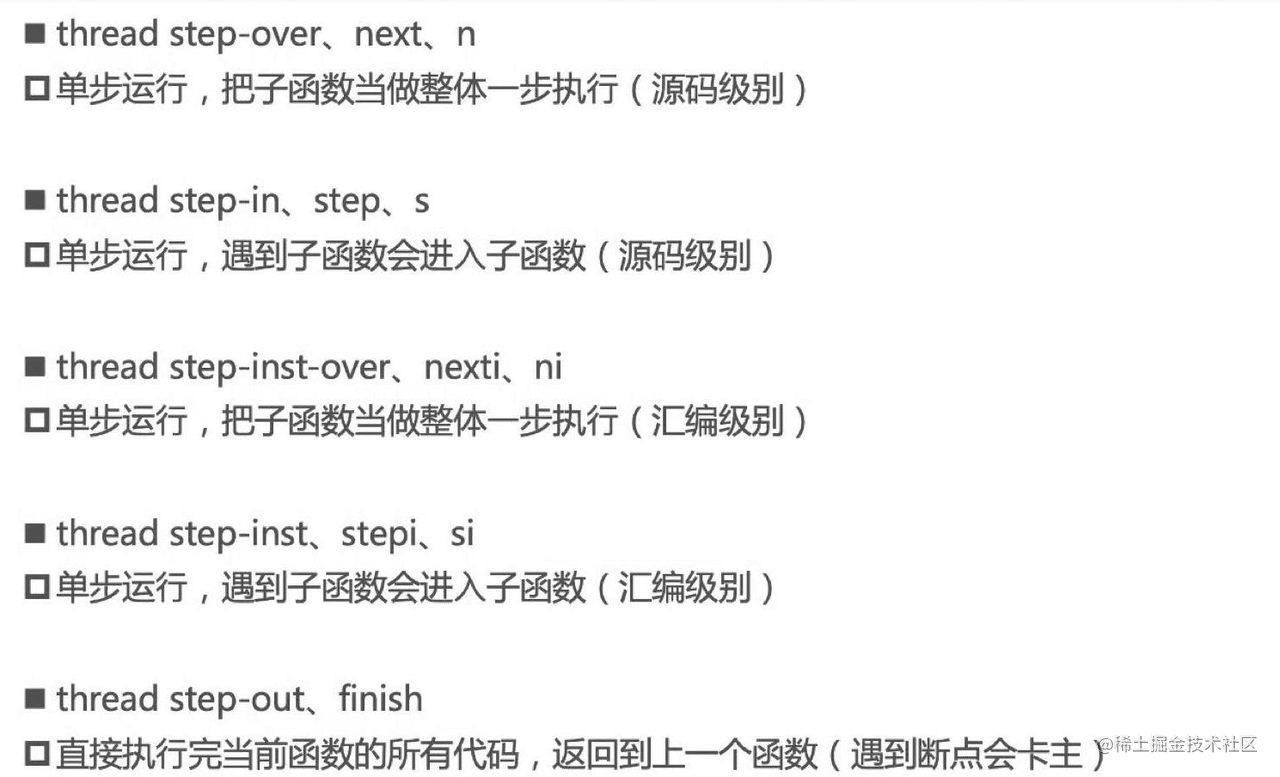## 三、彙編舉例

==3.1== 通過彙編，發現`sizeof()`的本質是編譯器關鍵字，而不是函式。 ```objc int main(int argc, const char * argv[]) { int c = sizeof(int); int b = 5; // 此處打斷點 return 0; }``` 打斷點進入彙編指令的截圖：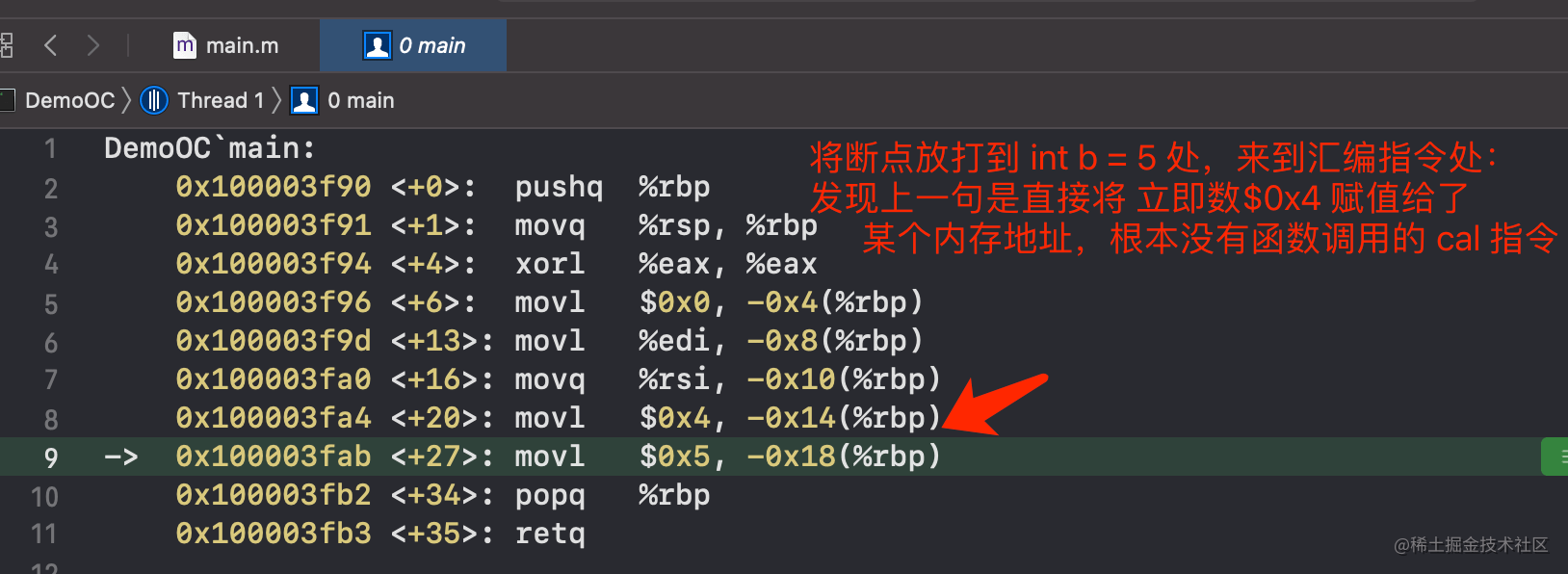==3.2== Swift裡面`inout`的執行彙編截圖： ```swift var a = 5 func test(_ number: inout Int) { number = 8 } test(&a) // 斷點處``` 執行，進入彙編指令頁面：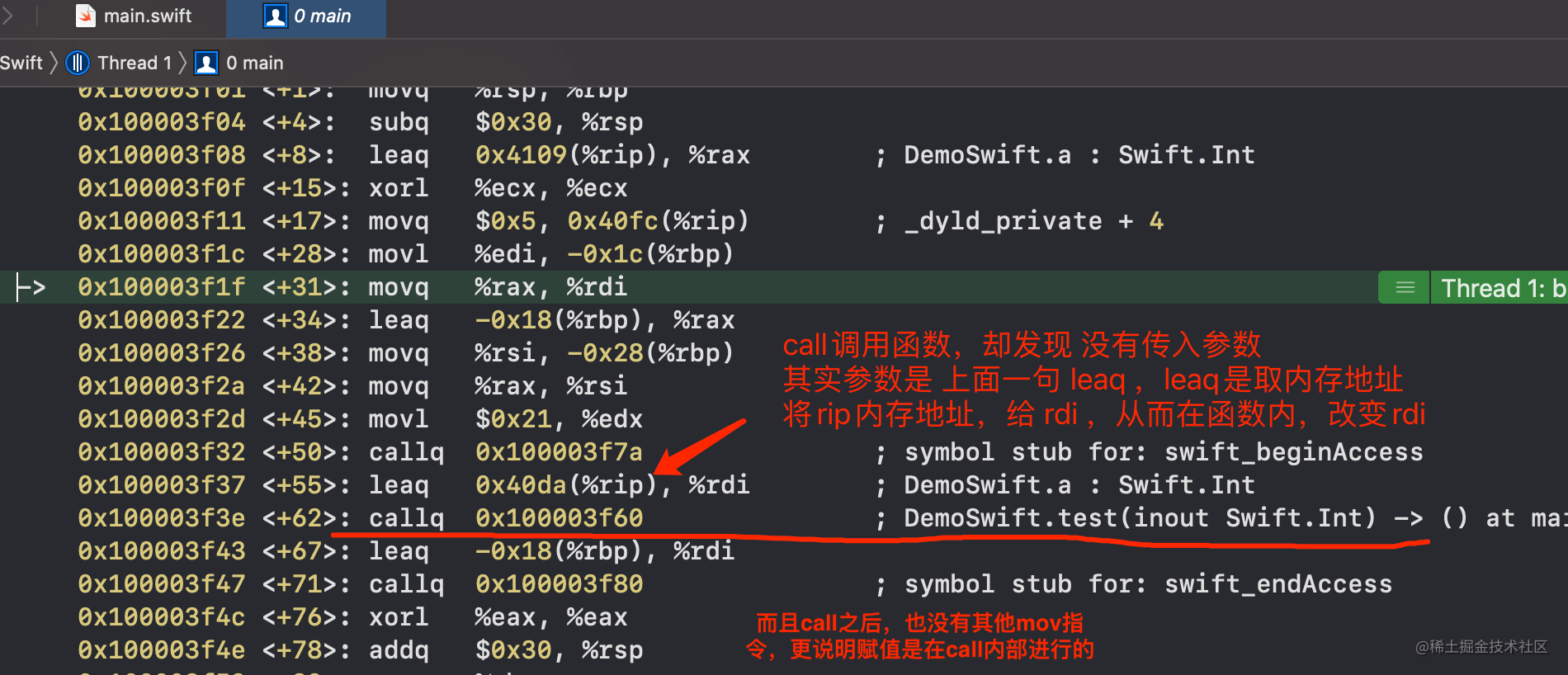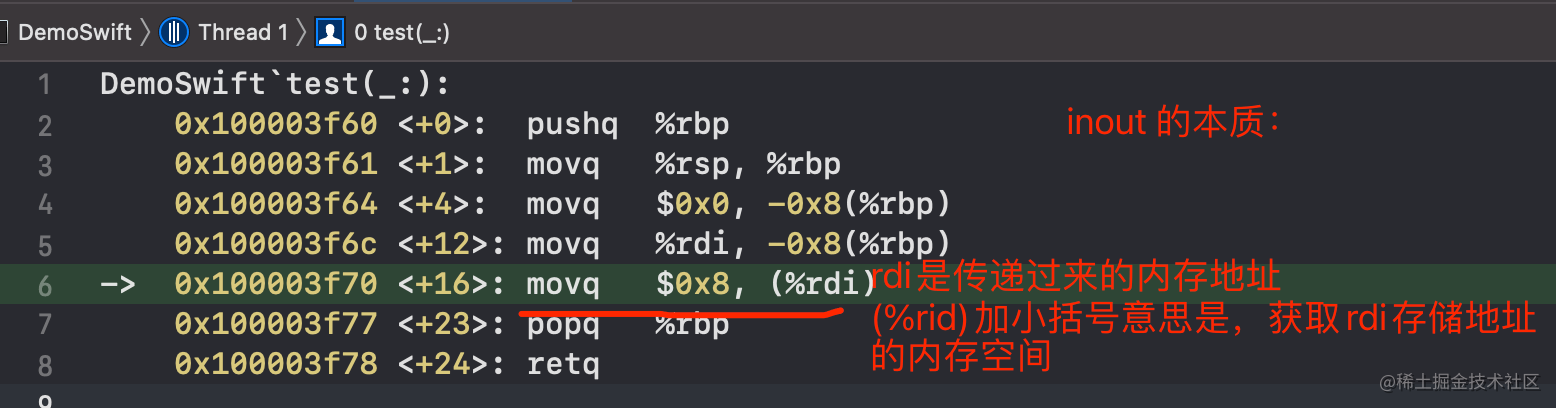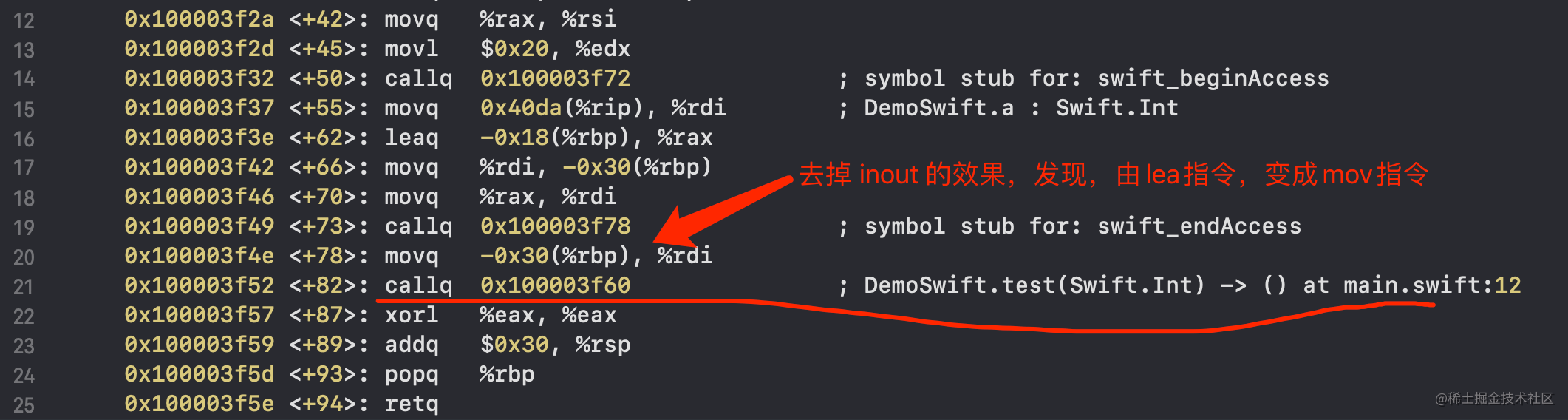`mov`指令直接將a的值，賦值給了形參。`lea`指令將a的記憶體地址傳遞到了函式內部。說明了`inout`的工作原理。

==3.3== Swift是有預設的main函式的，只是不用自己去寫，當我們進入斷點之後，能看到彙編頁面的頂部有main方法的入口： ```Delphi DemoSwift`main: // 進入彙編，看到main呼叫 0x100003f00 <+0>: pushq %rbp 0x100003f01 <+1>: movq %rsp, %rbp```

==3.4== 所有的函式(方法)不管有沒有返回值，編譯後，都會有`return`的，這也是`call`指令和`jump`指令的關鍵區別，程式碼演示：

```swift func testFunc() { let a = 5 let b = 6 let c = a + b } testFunc() let a = 5 // 斷點位置```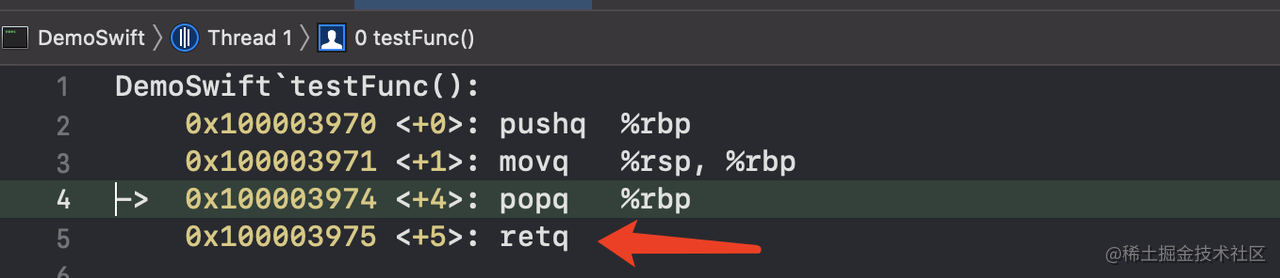• 從另外的角度，函式執行之後，需要回到呼叫函式的位置，所以必然有`ret`指令。
• `call`指令是呼叫函式，必然會有`ret`指令回來繼續執行。
• `jump`是直接跳到對應的指令執行，不會再回來。

## 四、Swift奇趣漫談

### 4.1 Swift的函式過載

1.返回值型別 和 函式過載無關，下面三個函式，同時存在，是可以編譯通過的。 ```swift func sum(v1: Int, v2: Int) -> Int { 1 } func sum(v1: Int, v2: Int) -> Double { 1.0 } func sum(v1: Int, v2: Int) { }```

// ❌報錯：Ambiguous use of 'sum(v1:v2:)' sum(v1: 10, v2: 20) ```

### 4.4 函式過載總結

func sum(_ v1: Int, _ v2: Int) -> Int { print("sum1") return v1 + v2 }

func sum(_ v1: Int, _ v2: Int, _ v3: Int = 10) -> Int { print("sum3") return v1 + v2 }

print( sum(10, 20) ) // 你猜會呼叫哪個函式？ ```

### 4.6 列舉的初始化

Swift的列舉是值型別，`struct`也是值型別，那麼二者有哪些區別呢？比如下面的程式碼： ```swift enum MyEnum { case test1(Int, Int, Int) case test2 }

struct MyStruct { let age: Int }

let e = MyEnum.test1(2, 3, 4) // 斷點處 let p = MyStruct(age: 5) ``` 執行進入斷點，在彙編指令頁面，我發現了神奇的對比：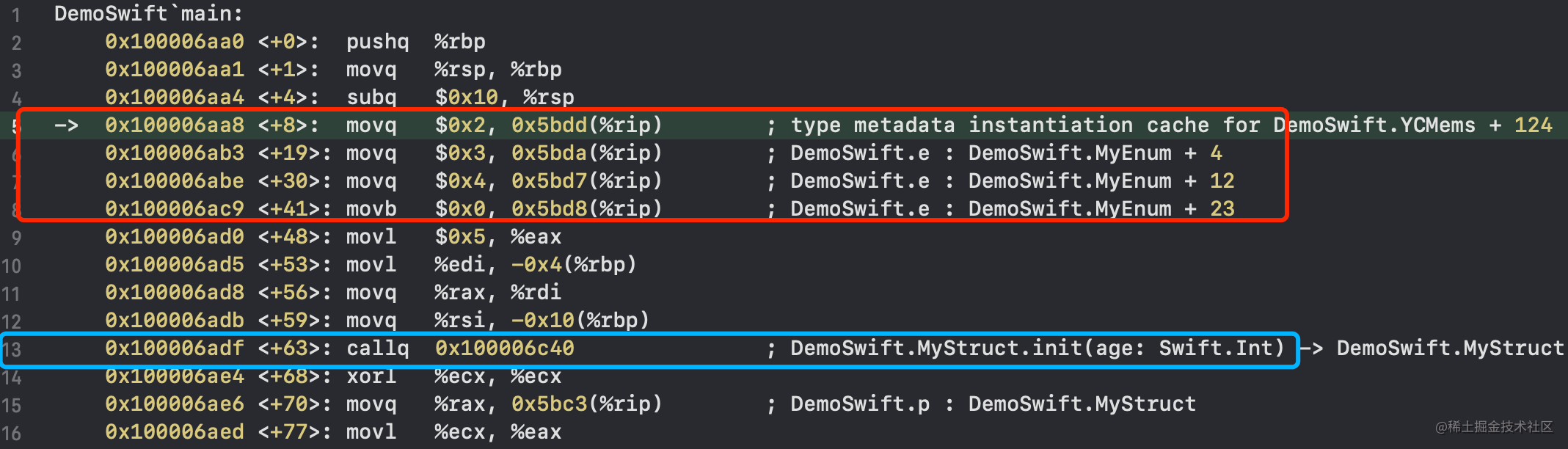### 4.7 列舉關聯值和原始值的記憶體區別

// 利用case模式，可以獲得關聯值，比如當是`Date.digit`型別，拿到year if case let .digit(yeaer: year, month: , day: ) = d1 { print(year) } ```

var pwd = Password.number(1, 2, 3, 4) pwd = .other

print( MemoryLayout.size(ofValue: pwd) ) // 33 實際用到的記憶體空間大小 print( MemoryLayout.stride(ofValue: pwd) ) // 40 系統分配佔用的空間大小，會記憶體對齊 print( MemoryLayout.alignment(ofValue: pwd) ) // 8 記憶體對齊引數

print("---------------")

// 2.原始值 enum Names: Int { case xiaoMing = 2, xiaoHong = 5, xiaoHou = 6, XiaoLi = 9 }

let name = Names.XiaoLi

print( MemoryLayout.size(ofValue: name) ) // 1 實際用到的記憶體空間大小 print( MemoryLayout.stride(ofValue: name) ) // 1 系統分配佔用的空間大小，會記憶體對齊 print( MemoryLayout.alignment(ofValue: name) ) // 1 記憶體對齊引數 ````` ==結論：== 原始值 是固定死的，可以通過程式碼```switch```判斷返回，所以不用佔儲存記憶體。 關聯值 是要要外面傳入的，是會變化的，必須得存起來，是會佔用記憶體的。 - 1個位元組儲存成員值 - N個位元組儲存關聯值（N取佔用記憶體最大的關聯值），任何一個```case`的關聯值都共用這N個位元組

enum TestEnum { case test1, test2, test3 } var t = TestEnum.test1 print(memPtr(ofValue: &t)) // 列印記憶體地址，複製貼上到`View Member`中檢視記憶體 print("--------我是分割線，為了方便斷點--------")

t = .test2 print("--------我是分割線，為了方便斷點--------")

t = .test3 print("--------我是分割線，為了方便斷點--------") ```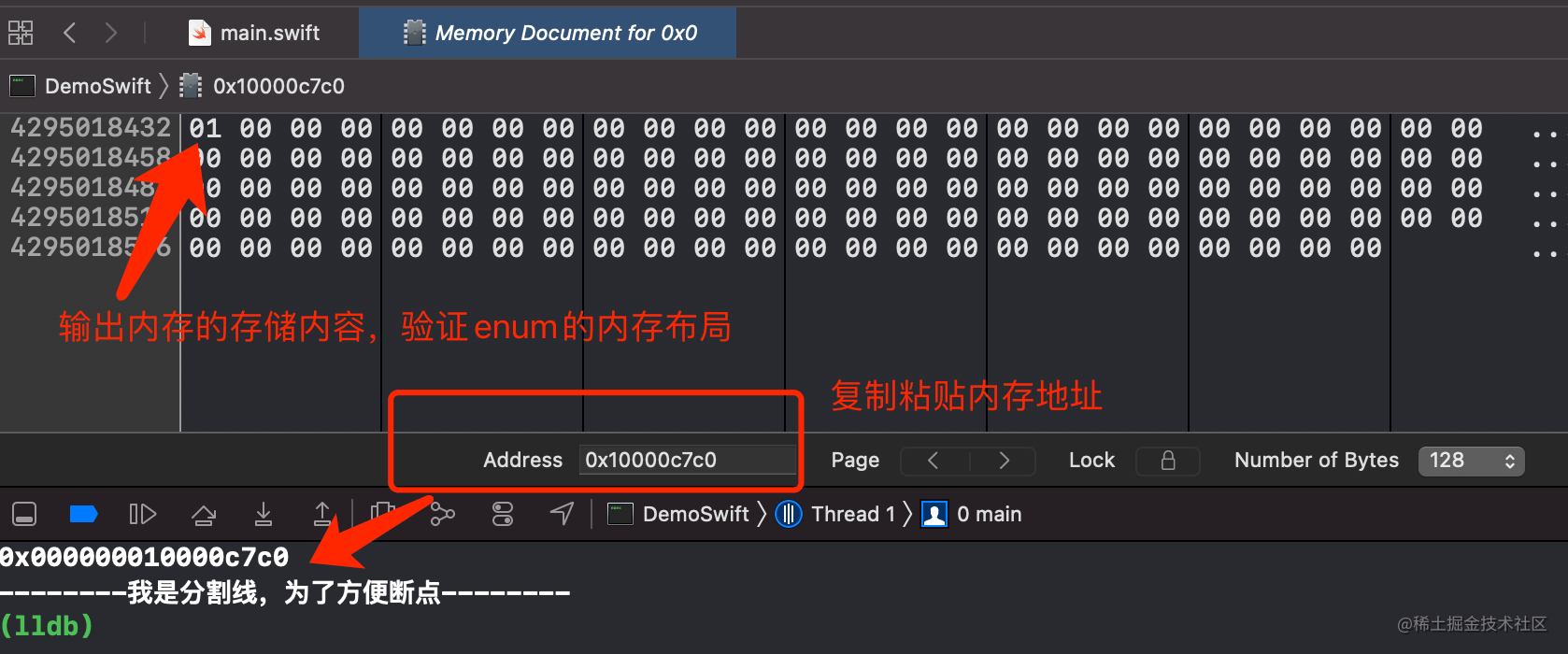var t = TestEnum.test1(5, 6, 7) print(memPtr(ofValue: &t)) print("--------我是分割線，為了方便斷點--------")

t = .test2(8, 9) print("--------我是分割線，為了方便斷點--------") ```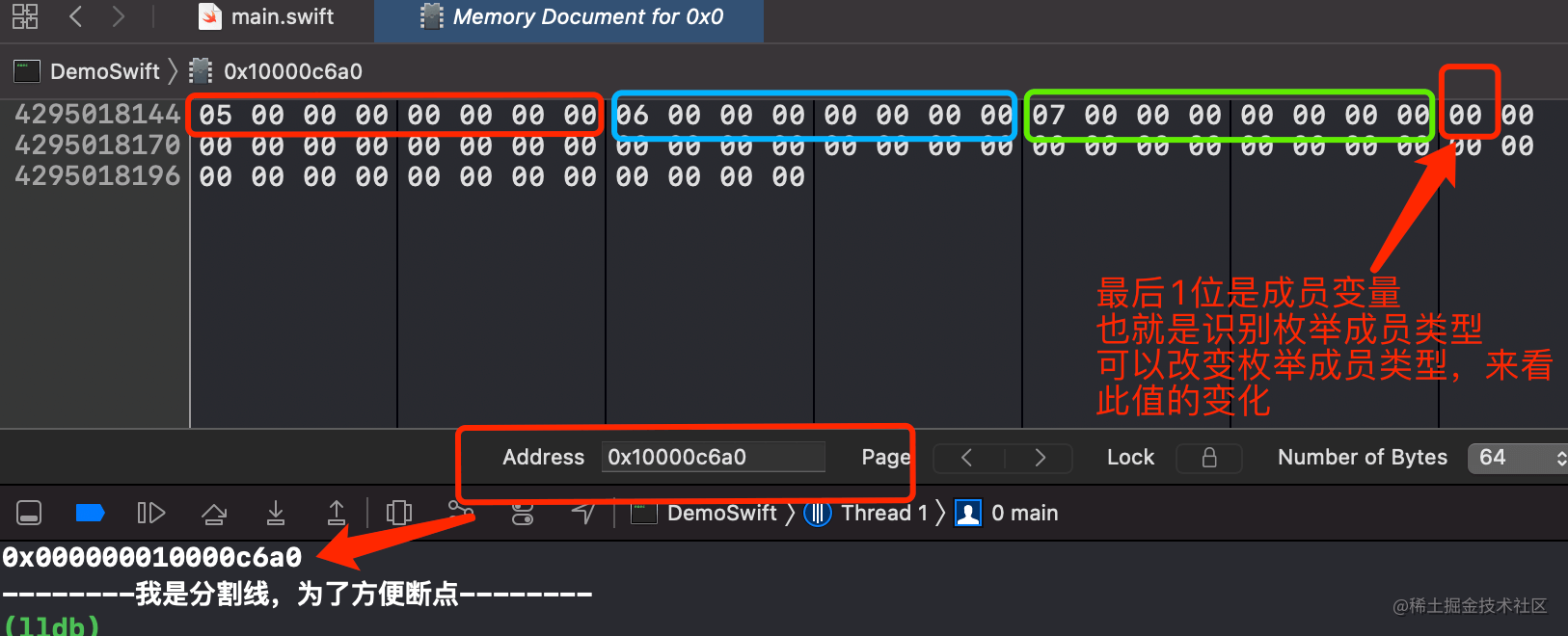### 4.8 ??和if-let配合使用

let a: Int? = nil let b: Int? = 2 if let c = a ?? b { // 類似於 if a != nil || b != nil print(c) } if let _ = a ?? b { } // 或者這種奇怪的程式碼 ```

==結論：== `??`返回值類的型別，就看 最後一個`??`後面的變數型別，和後一個變數型別一致。

### 4.9 多重可選項

// 下面分別輸出什麼？ print(num2 == num3) print( (num2 ?? 1) ?? 2 ) print( (num3 ?? 1) ?? 2 ) ```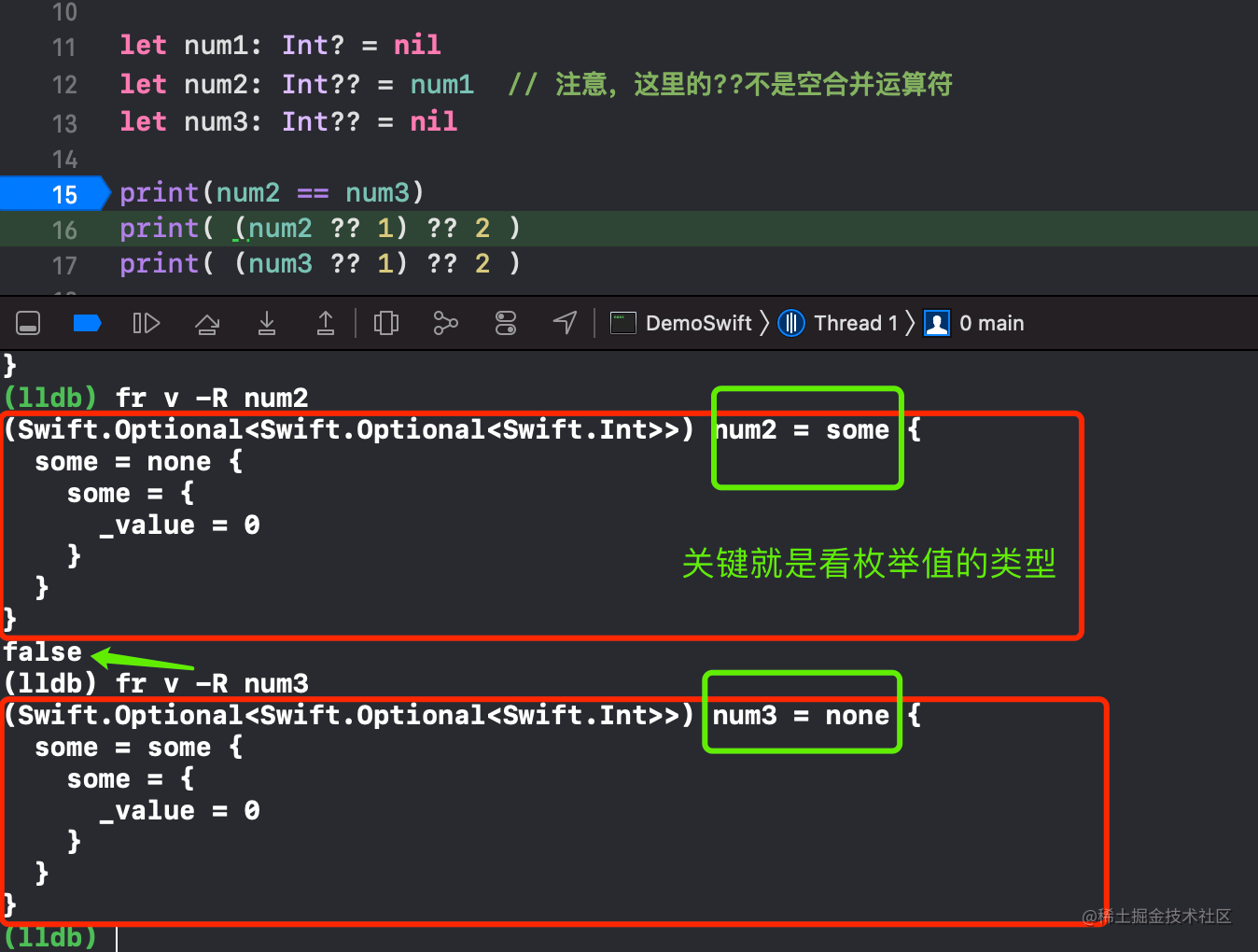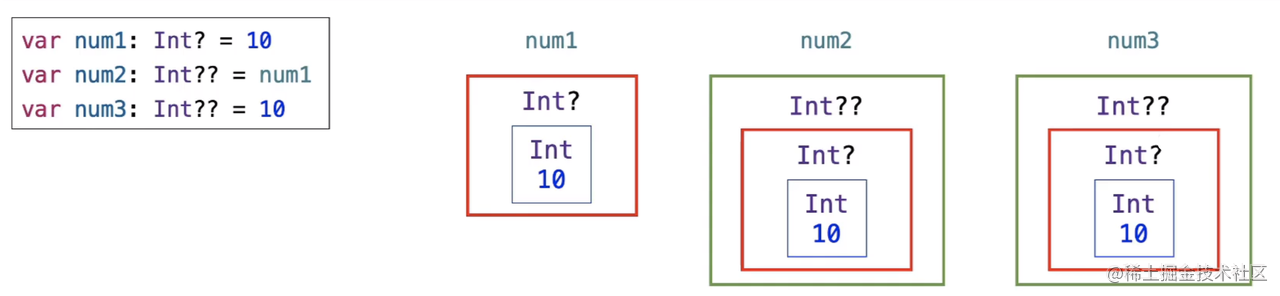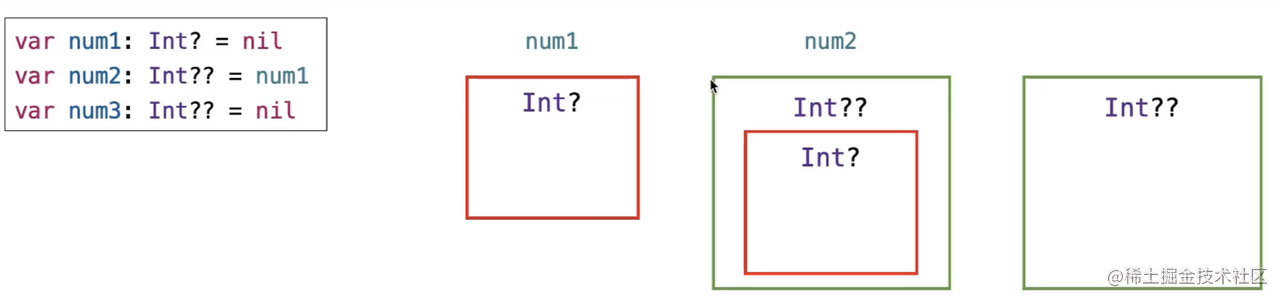### 4.10 結構體初始化器的下面2種寫法，是否一樣？

```swift struct Point { var x: Int = 5 var y: Int = 6 }

struct Point { var x: Int var y: Int

init() { // 寫出來了init() x = 5 y = 6 } } ```

### 4.11 Swift類的初始化過程

```swift class Person { let age: Int init(age: Int) { self.age = age } }

let p = Person(age: 18) // 斷點 ```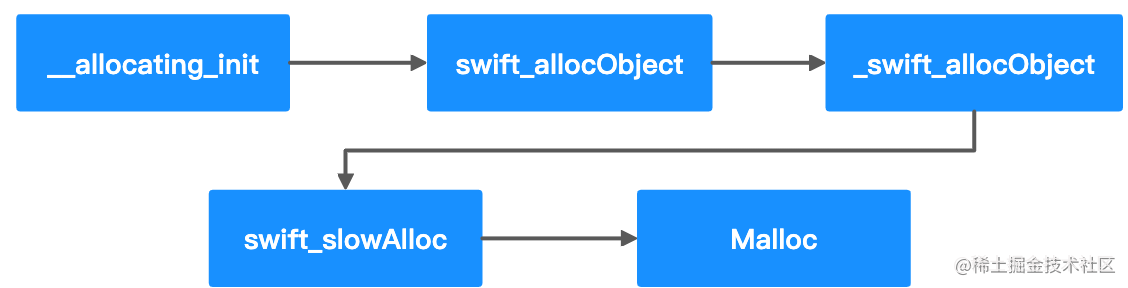### 4.12 Swift的超類

print( class_getInstanceSize(Person.self) ) // 16 print( class_getSuperclass(Student.self)! ) // Persong print( class_getSuperclass(Person.self)! ) // _TtCs12_SwiftObject ```

END。

「其他文章」